# Resources tagged with: Triangles

Filter by: Content type:
Age range:
Challenge level:

### There are 57 results

Broad Topics > Angles, Polygons, and Geometrical Proof > Triangles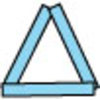### Sticks and Triangles

##### Age 7 to 11 Challenge Level:

Using different numbers of sticks, how many different triangles are you able to make? Can you make any rules about the numbers of sticks that make the most triangles?### Egyptian Rope

##### Age 7 to 11 Challenge Level:

The ancient Egyptians were said to make right-angled triangles using a rope with twelve equal sections divided by knots. What other triangles could you make if you had a rope like this?### More Transformations on a Pegboard

##### Age 7 to 11 Challenge Level:

Use the interactivity to find all the different right-angled triangles you can make by just moving one corner of the starting triangle.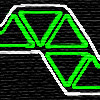### Tri-five

##### Age 7 to 11 Challenge Level:

Find all the different shapes that can be made by joining five equilateral triangles edge to edge.### Uncanny Triangles

##### Age 7 to 11 Challenge Level:

Can you help the children find the two triangles which have the lengths of two sides numerically equal to their areas?### Tri.'s

##### Age 7 to 11 Challenge Level:

How many triangles can you make on the 3 by 3 pegboard?### Putting Two and Two Together

##### Age 7 to 11 Challenge Level:

In how many ways can you fit two of these yellow triangles together? Can you predict the number of ways two blue triangles can be fitted together?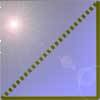### Cutting Corners

##### Age 7 to 11 Challenge Level:

Can you make the most extraordinary, the most amazing, the most unusual patterns/designs from these triangles which are made in a special way?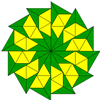### Triangle Shapes

##### Age 5 to 11 Challenge Level:

This practical problem challenges you to create shapes and patterns with two different types of triangle. You could even try overlapping them.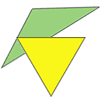### Triangle Relations

##### Age 7 to 11 Challenge Level:

What do these two triangles have in common? How are they related?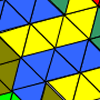### Tessellating Transformations

##### Age 7 to 11 Challenge Level:

Can you find out how the 6-triangle shape is transformed in these tessellations? Will the tessellations go on for ever? Why or why not?### Cut it Out

##### Age 7 to 11 Challenge Level:

Can you dissect an equilateral triangle into 6 smaller ones? What number of smaller equilateral triangles is it NOT possible to dissect a larger equilateral triangle into?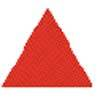### Tessellating Triangles

##### Age 7 to 11 Challenge Level:

Can you make these equilateral triangles fit together to cover the paper without any gaps between them? Can you tessellate isosceles triangles?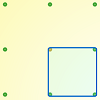### Peg and Pin Boards

##### Age 5 to 11

This article for teachers suggests activities based on pegboards, from pattern generation to finding all possible triangles, for example.### Inside Seven Squares

##### Age 7 to 11 Challenge Level:

What is the total area of the four outside triangles which are outlined in red in this arrangement of squares inside each other?### Triangle Pin-down

##### Age 7 to 11 Challenge Level:

Use the interactivity to investigate what kinds of triangles can be drawn on peg boards with different numbers of pegs.### Nine-pin Triangles

##### Age 7 to 11 Challenge Level:

How many different triangles can you make on a circular pegboard that has nine pegs?### Bracelets

##### Age 7 to 11 Challenge Level:

Investigate the different shaped bracelets you could make from 18 different spherical beads. How do they compare if you use 24 beads?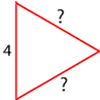### Number the Sides

##### Age 7 to 11 Challenge Level:

The triangles in these sets are similar - can you work out the lengths of the sides which have question marks?### Cut and Make

##### Age 7 to 11 Challenge Level:

Cut a square of paper into three pieces as shown. Now,can you use the 3 pieces to make a large triangle, a parallelogram and the square again?### Folding

##### Age 7 to 11 Challenge Level:

What shapes can you make by folding an A4 piece of paper?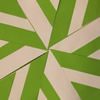### Folding Flowers 2

##### Age 7 to 11 Challenge Level:

Make a flower design using the same shape made out of different sizes of paper.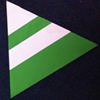### Regular Rings 1

##### Age 7 to 11 Challenge Level:

Can you work out what shape is made by folding in this way? Why not create some patterns using this shape but in different sizes?### Transformations on a Pegboard

##### Age 7 to 11 Challenge Level:

How would you move the bands on the pegboard to alter these shapes?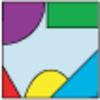### Jig Shapes

##### Age 5 to 11 Challenge Level:

Can you each work out what shape you have part of on your card? What will the rest of it look like?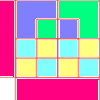### Diagrams

##### Age 7 to 11 Challenge Level:

A group activity using visualisation of squares and triangles.### Cutting it Out

##### Age 5 to 11 Challenge Level:

I cut this square into two different shapes. What can you say about the relationship between them?### Triangles All Around

##### Age 7 to 11 Challenge Level:

Can you find all the different triangles on these peg boards, and find their angles?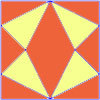### Fraction Fascination

##### Age 7 to 11 Challenge Level:

This problem challenges you to work out what fraction of the whole area of these pictures is taken up by various shapes.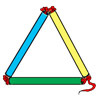### Making Maths: Test the Strength of a Triangle

##### Age 7 to 11 Challenge Level:

Have you noticed that triangles are used in manmade structures? Perhaps there is a good reason for this? 'Test a Triangle' and see how rigid triangles are.### Name That Triangle!

##### Age 7 to 11 Challenge Level:

Can you sketch triangles that fit in the cells in this grid? Which ones are impossible? How do you know?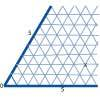### Cartesian Isometric

##### Age 7 to 11 Challenge Level:

The graph below is an oblique coordinate system based on 60 degree angles. It was drawn on isometric paper. What kinds of triangles do these points form?### Constructing Triangles

##### Age 11 to 14 Challenge Level:

Generate three random numbers to determine the side lengths of a triangle. What triangles can you draw?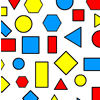### Sorting Logic Blocks

##### Age 5 to 11 Challenge Level:

This interactivity allows you to sort logic blocks by dragging their images.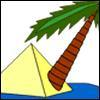### Triangle Island

##### Age 7 to 11 Challenge Level:

You have pitched your tent (the red triangle) on an island. Can you move it to the position shown by the purple triangle making sure you obey the rules?### Possible Pairs

##### Age 7 to 11 Challenge Level:

In this game, you turn over two cards and try to draw a triangle which has both properties.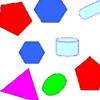### Part the Polygons

##### Age 7 to 11 Short Challenge Level:

Draw three straight lines to separate these shapes into four groups - each group must contain one of each shape.### Rectangle Tangle

##### Age 7 to 11 Challenge Level:

The large rectangle is divided into a series of smaller quadrilaterals and triangles. Can you untangle what fractional part is represented by each of the shapes?### Isosceles Triangles

##### Age 11 to 14 Challenge Level:

Draw some isosceles triangles with an area of $9$cm$^2$ and a vertex at (20,20). If all the vertices must have whole number coordinates, how many is it possible to draw?### Tetrahedra Tester

##### Age 11 to 14 Challenge Level:

An irregular tetrahedron is composed of four different triangles. Can such a tetrahedron be constructed where the side lengths are 4, 5, 6, 7, 8 and 9 units of length?### Ratty

##### Age 11 to 14 Challenge Level:

If you know the sizes of the angles marked with coloured dots in this diagram which angles can you find by calculation?### Dotty Triangles

##### Age 11 to 14 Challenge Level:

Imagine an infinitely large sheet of square dotty paper on which you can draw triangles of any size you wish (providing each vertex is on a dot). What areas is it/is it not possible to draw?### Property Chart

##### Age 11 to 14 Challenge Level:

A game in which players take it in turns to try to draw quadrilaterals (or triangles) with particular properties. Is it possible to fill the game grid?### Shapely Pairs

##### Age 11 to 14 Challenge Level:

A game in which players take it in turns to turn up two cards. If they can draw a triangle which satisfies both properties they win the pair of cards. And a few challenging questions to follow...### Counting Triangles

##### Age 11 to 14 Challenge Level:

Triangles are formed by joining the vertices of a skeletal cube. How many different types of triangle are there? How many triangles altogether?### Triangle Transformation

##### Age 7 to 14 Challenge Level:

Start with a triangle. Can you cut it up to make a rectangle?### Making Maths: Equilateral Triangle Folding

##### Age 7 to 14 Challenge Level:

Make an equilateral triangle by folding paper and use it to make patterns of your own.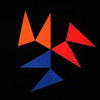### Notes on a Triangle

##### Age 11 to 14 Challenge Level:

Can you describe what happens in this film?### Triangular Tantaliser

##### Age 11 to 14 Challenge Level:

Draw all the possible distinct triangles on a 4 x 4 dotty grid. Convince me that you have all possible triangles.### Trice

##### Age 11 to 14 Challenge Level:

ABCDEFGH is a 3 by 3 by 3 cube. Point P is 1/3 along AB (that is AP : PB = 1 : 2), point Q is 1/3 along GH and point R is 1/3 along ED. What is the area of the triangle PQR?